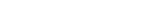2787 is valid Arabic numeral. Here we will explain how to read, write and convert the Arabic numeral 2787 into the correct Roman numeral format. Please have a look over the Roman numeral table given below for better understanding of Roman numeral system. As you can see, each letter is associated with specific value.

Symbol Value
I1
V5
X10
L50
C100
D500
M1000

# How to write Arabic Numeral 2787 in Roman numeral?

The Roman numeral representation of Arabic numeral 2787 is MMDCCLXXXVII.

# How to convert Arabic numeral 2787 to Roman numeral?

If you are aware of Roman numeral system, then converting Arabic numeral 2787 to Roman numeral is very easy. Converting 2787 to Roman numeral representation involves breaking up the numeral into place values as shown below.

 2787 1000 + 1000 + 500 + 100 + 100 + 50 + 10 + 10 + 10 + 5 + 1 + 1 M + M + D + C + C + L + X + X + X + V + I + I MMDCCLXXXVII

We need to combine all converted roman numerals together. As per the rule highest numeral should always precede the lowest numeral to get correct representation. In modern world, the number 2787 should be used as it is until or unless it represents an ordinal value. In case of any ordinal value, you can use MMDCCLXXXVII instead of 2787. For any numeral conversion, you can also use our roman to number converter tool given above.

Disclaimer:We make a reasonable effort in making sure that conversion results are as accurate as possible, but we cannot guarantee that. Before using any details provided here, you must validate its correctness from other reliable sources on internet.